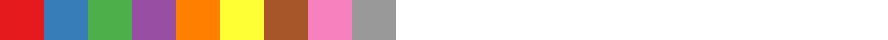# @vx/scale

``````npm install --save @vx/scale
``````

## Overview of Scaling

The `@vx/scale` package aims to provide a wrapper around existing d3 scaling originally defined in the d3-scale package.

Scales are functions that help you map your data to the physical pixel size that your graph requires. For example, let's say you wanted to create a bar chart to show populations per country. If you were to use a 1-to-1 scale (IE: 1 pixel per y value) your bar for the USA would be about 321.4 million pixels high!

Instead, you can tell vx a function to use that takes a value (like your population per country) and spits out another value.

For example, we could create a linear scale like this:

``````const graphHeight = 500; // We'll have a 500 pixel high graph
const maxPopulation = getMostPopulatedCountryInTheWorld();

const yScale = Scale.scaleLinear({
rangeRound: [graphHeight, 0],
domain: [0, maxPopulation],
});

// ...

const bars = data.map((d, i) => {
const barHeight = graphHeight - yScale(d.y);
return <Shape.Bar height={barHeight} y={graphHeight - barHeight} />
})
``````

Note: This example represents how to use a yScale, but skipped a lot of details about how to make a bar chart. If you're trying to do that, you should check out this example.

## Current Scaling Options

### Band Scaling

Original d3 docs

Example:

``````const scale = Scale.scaleBand({
/*
range,
rangeRound,
domain,
padding,
nice = false
*/
});
``````

### Linear Scaling

Original d3 docs

Example:

``````const scale = Scale.scaleLinear({
/*
range,
rangeRound,
domain,
nice = false,
clamp = false,
*/
});
``````

### Log Scaling

Original d3 docs

Example:

``````const scale = Scale.scaleLog({
/*
range,
rangeRound,
domain,
base,
nice = false,
clamp = false,
*/
});
``````

### Ordinal Scaling

Original d3 docs

Example:

``````const scale = Scale.scaleOrdinal({
/*
range,
domain,
unknown,
*/
});
``````

### Point Scaling

Original d3 docs

Example:

``````const scale = Scale.scalePoint({
/*
range,
rangeRound,
domain,
padding,
align,
nice = false,
*/
});
``````

### Power Scaling

Original d3 docs

Example:

``````const scale = Scale.scalePower({
/*
range,
rangeRound,
domain,
exponent,
nice = false,
clamp = false,
*/
});
``````

### Time Scaling

Original d3 docs

Example:

``````const scale = Scale.scaleTime({
/*
range,
rangeRound,
domain,
nice = false,
clamp = false,
*/
});
``````

You also can scale time with Coordinated Universal Time via `scaleUtc`.

Example:

``````const scale = Scale.scaleUtc({
/*
range,
rangeRound,
domain,
nice = false,
clamp = false,
*/
});
``````

### Color Scales

D3 scales offer the ability to map points to colors. You can use `d3-scale-chromatic` in conjunction with vx's `scaleOrdinal` to make color scales.

You can install `d3-scale-chromatic` with npm:

``````npm install --save d3-scale-chromatic
``````

You create a color scale like so:

``````import { scaleOrdinal } from '@vx/scale';
import { schemeSet1 } from 'd3-scale-chromatic';

const colorScale = scaleOrdinal({
domain: arrayOfThings,
range: schemeSet1
});
``````

This generates a color scale with the following colors:There are a number of other categorical color schemes available, along with other continuous color schemes.